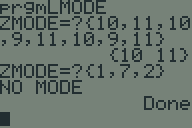## Multinomial Probability Mass Function in TI-BASICAnother TI-BASIC challenge proposed to me by the same person who asked me last time was to calculate the probability mass function of a multinomial distribution. The formula is:

$$\frac{n!}{x_1 \cdots x_k} p_1^{x_1}\cdots p_k^{x_k} \mbox{ where } n = \sum_{i=1}^k x_i$$
The naïve way of calculating this is to read $x_1$ and $p_1$ through $x_k$ and $p_k$ and then crunch the numbers. However, this necessitates a list to keep track of all the numbers and an extra loop at the end to crunch them. Since we don’t actually need the numbers, there’s an easier way.

## Calculating the mode of a list in TI-BASICRecently, someone challenged me to write a program to find the mode (or modes) of a list in TI-BASIC. I picked up my TI-83+ and whipped this program up in about half an hour. Somebody else asked me for a copy, so I figured I’d post it on my website.